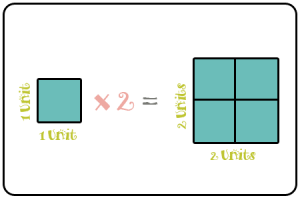Select Page

We’ve done many projects over the course of the past two years. One of the favorites of the students though was the Angry Birds posters we created last year. While learning about angles, students were asked to create their own Angry Birds level. They then had to use protractors to measure the launch angles of each bird. It was a fun way to learn about how to measure an angle!

When we created those poster last year, Mr. Avery was teaching fourth grade. Now teaching sixth grade, we wanted to do the Angry Bird posters but we needed to add something else to them to tie in to what we were currently learning about. So, this year, instead of just measuring angles, we also used it to learn about proportions!

A proportion is another term that refers to similar figures. Students had previously learned that similar figures have the same shape but are not the same size. You can always tell if figures are proportional to each other based upon whether they are similar.

Sometimes we have to create proportional shapes. We can do so by measuring the shape that we are given and then multiplying or dividing the sides by the same number. For example, in the picture below, our first square is one unit by one unit. If we multiply the sides by 2, our proportional square becomes 2 units by 2 units.You could also multiply your sides by 4 and have a square that is 4 units by 4 units. You could multiply your sides by 10 and have a square that is 10 units by 10 units. You could even multiply it by 1,000 and you would have a square that is 1,000 units by 1,000 units! All of those squares would be proportional to our original shape because the figures are similar. They’re the same shape, just different sizes!

For our project, students paired up and then because of the time of year, they were asked to design an Angry Bird level based on the Angry Bird Seasons game. Students started out with a 15 unit by 12 unit grid. They had to sketch the layout of their design.

After students figured out their designs, it was time to create our actual posters. The measurement of the posters was 30 units by 24 units, twice the size of the original grid. Students had to double the size of everything in their layout for their final product! In doing so, the poster was proportional to the original sketch.

We still kept the angle aspect of the project as well. After the posters were completed, students had to use a compass to show the trajectory of each bird. We then used a protractor to show the angle that each bird would have to be launched from the slingshot to complete the level.

Take a look at our finished product in the slideshow below!

*Where might proportions come in handy outside of school?*

*What was your favorite game you played growing up?*